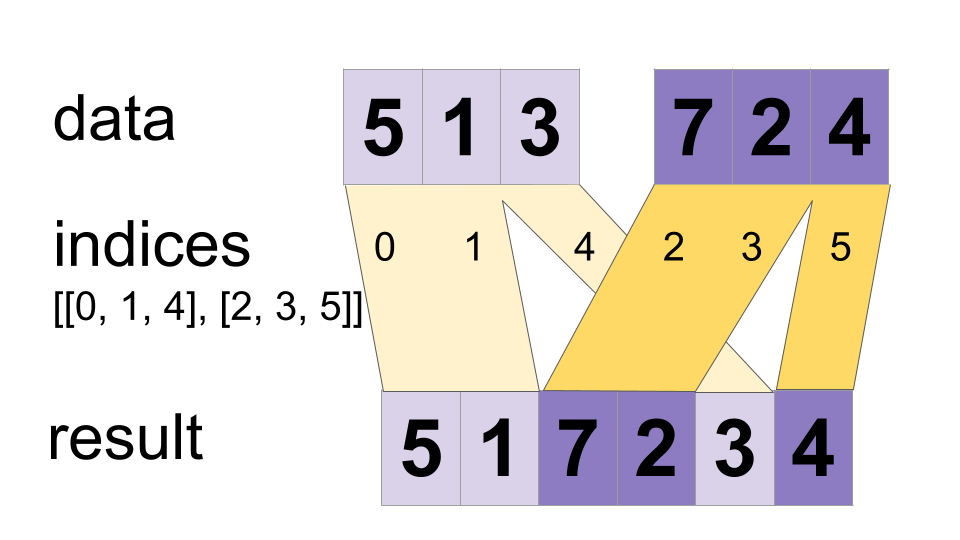Have a question? Connect with the community at the TensorFlow Forum

# tf.dynamic_stitch

Interleave the values from the `data` tensors into a single tensor.

Builds a merged tensor such that

``````    merged[indices[m][i, ..., j], ...] = data[m][i, ..., j, ...]
``````

For example, if each `indices[m]` is scalar or vector, we have

``````    # Scalar indices:
merged[indices[m], ...] = data[m][...]

# Vector indices:
merged[indices[m][i], ...] = data[m][i, ...]
``````

Each `data[i].shape` must start with the corresponding `indices[i].shape`, and the rest of `data[i].shape` must be constant w.r.t. `i`. That is, we must have `data[i].shape = indices[i].shape + constant`. In terms of this `constant`, the output shape is

``````merged.shape = [max(indices)] + constant
``````

Values are merged in order, so if an index appears in both `indices[m][i]` and `indices[n][j]` for `(m,i) < (n,j)` the slice `data[n][j]` will appear in the merged result. If you do not need this guarantee, ParallelDynamicStitch might perform better on some devices.

#### For example:

``````    indices = 6
indices = [4, 1]
indices = [[5, 2], [0, 3]]
data = [61, 62]
data = [[41, 42], [11, 12]]
data = [[[51, 52], [21, 22]], [[1, 2], [31, 32]]]
merged = [[1, 2], [11, 12], [21, 22], [31, 32], [41, 42],
[51, 52], [61, 62]]
``````

This method can be used to merge partitions created by `dynamic_partition` as illustrated on the following example:

``````    # Apply function (increments x_i) on elements for which a certain condition
# apply (x_i != -1 in this example).
x=tf.constant([0.1, -1., 5.2, 4.3, -1., 7.4])
condition_mask=tf.not_equal(x,tf.constant(-1.))
partitioned_data = tf.dynamic_partition(
x, tf.cast(condition_mask, tf.int32) , 2)
partitioned_data = partitioned_data + 1.0
condition_indices = tf.dynamic_partition(
tf.range(tf.shape(x)), tf.cast(condition_mask, tf.int32) , 2)
x = tf.dynamic_stitch(condition_indices, partitioned_data)
# Here x=[1.1, -1., 6.2, 5.3, -1, 8.4], the -1. values remain
# unchanged.
```````indices` A list of at least 1 `Tensor` objects with type `int32`.
`data` A list with the same length as `indices` of `Tensor` objects with the same type.
`name` A name for the operation (optional).

A `Tensor`. Has the same type as `data`.

[{ "type": "thumb-down", "id": "missingTheInformationINeed", "label":"Missing the information I need" },{ "type": "thumb-down", "id": "tooComplicatedTooManySteps", "label":"Too complicated / too many steps" },{ "type": "thumb-down", "id": "outOfDate", "label":"Out of date" },{ "type": "thumb-down", "id": "samplesCodeIssue", "label":"Samples / code issue" },{ "type": "thumb-down", "id": "otherDown", "label":"Other" }]
[{ "type": "thumb-up", "id": "easyToUnderstand", "label":"Easy to understand" },{ "type": "thumb-up", "id": "solvedMyProblem", "label":"Solved my problem" },{ "type": "thumb-up", "id": "otherUp", "label":"Other" }]